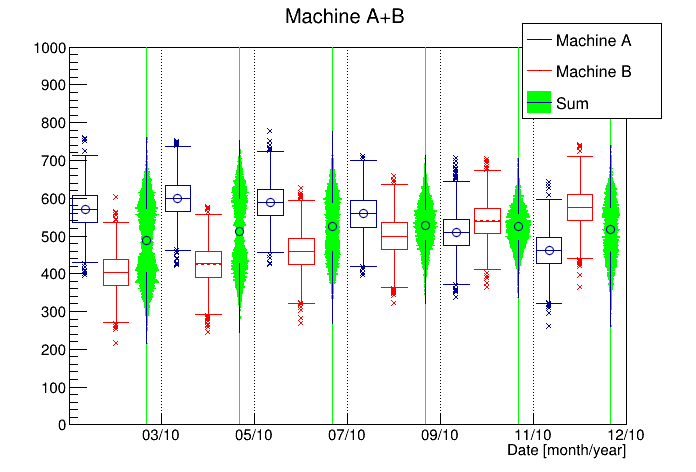# Candleplotstack¶

Example showing how a THStack with candle plot option.

Author: Georg Troska, Olivier Couet
This notebook tutorial was automatically generated with ROOTBOOK-izer from the macro found in the ROOT repository on Thursday, May 19, 2022 at 08:09 AM.

In :
gStyle->SetTimeOffset(0);
TRandom *rng       = new TRandom();
TDatime *dateBegin = new TDatime(2010,1,1,0,0,0);
TDatime *dateEnd   = new TDatime(2011,1,1,0,0,0);
int bins = 1000;
TH2I *h1 = new TH2I("h1","Machine A",6,dateBegin->Convert(),dateEnd->Convert(),bins,0,1000);
TH2I *h2 = new TH2I("h2","Machine B",6,dateBegin->Convert(),dateEnd->Convert(),bins,0,1000);
TH2I *hsum = new TH2I("h4","Sum",6,dateBegin->Convert(),dateEnd->Convert(),bins,0,1000);

float Rand;
for (int i = dateBegin->Convert(); i < dateEnd->Convert(); i+=86400*30) {
for (int j = 0; j < 1000; j++) {
Rand = rng->Gaus(500+sin(i/10000000.)*100,50); h1->Fill(i,Rand); hsum->Fill(i,Rand);
Rand = rng->Gaus(500+sin(i/12000000.)*100,50); h2->Fill(i,Rand); hsum->Fill(i,Rand);
}
}

h2->SetLineColor(kRed);
hsum->SetFillColor(kGreen);
TCanvas *c1 = new TCanvas();

THStack *hs = new THStack("hs","Machine A+B");
hs->Draw("candle3");
hs->GetXaxis()->SetNdivisions(410);

hs->GetXaxis()->SetTimeDisplay(1);
hs->GetXaxis()->SetTimeFormat("%m/%y");
hs->GetXaxis()->SetTitle("Date [month/year]");

c1->Modified();


gROOT->GetListOfCanvases()->Draw()